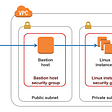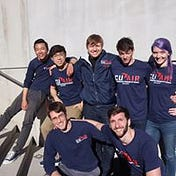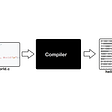# The Learning With Errors (LWE) Problem

• We view Z_q as integers in range (-q/2, q/2) as a finite field.
• We define ||e||_infinityas the infinity norm operation on vector e with m elements. The operations returns the element in vector e that has the greatest absolute value. Namely:
• Finally, we define x_B as a random variable that has its distribution bounded by a number B. Namely, every sample of this random variable will definitely be smaller than B.
• n is commonly referred to as the security parameter (\lambda). Therefore, the more unknown variables we have in the system, the harder the LWE problem becomes.
• m is usually a polynomial of n. Namely m = poly(n) and m >> n. The larger m goes, the easier the LWE problem gets.
• q is usually poly(n). For example, we can set q to be O(n²).
• B << q, we want the error bound to be negligible to the size of q, so we can properly recover the unknown vector.

# Putting it all together: Regev Encryption

1. KeyGen(1^\lambda): The KeyGen algorithm will first create an LWE problem instance. Namely, it will sample A <- Z_q^{m x n}, s <- Z_q^n, e <- x_B^m and set the product with error term to be b = As + e. We also need to enforce that q/4 > mB for the correctness property. Finally, it sets the private key sk = s, and the public key pk = (A, b).
2. Encrypt(pk, x = {0, 1}): The encryption algorithm encrypts a single bit at a time. Just like ElGamal, it will first sample some random binary nonce vector r <- Z_2^m \in {0,1}^m , then it will set the first part of the ciphertext c_0 to be r^T * A, and the second part of the ciphertext c_1 to be r^T * b + floor(q/2) x. Finally, it outputs both parts (c_0, c_1) as the final ciphertext.
3. Decrypt(sk, ct=(c_0, c_1)): To decrypt the ciphertext, first we compute x~ = c_1 — c_0 * s. Then we will check whether |x~| < q/4. If so, we output x = 0, else we output x = 1.

# Credits

## More from Steven Yue

Software Engineer & Part-time student. I code and do photography. Instagram: stevenyue. https://higashi.tech

Love podcasts or audiobooks? Learn on the go with our new app.

## Team Aladdin — A Carpet Ride into Machine Learning## BOOM: The First Self-Destructing NFT## Using Brakeman to secure your Rails app## SSL Implementation: PAID v/s FREE## Introduction Of The First Phase Of Matrix Blind Box## New Sauce for Sashimi, Community Governance Platform Launched## Connecting with SSH through a bastion server## Steven Yue

Software Engineer & Part-time student. I code and do photography. Instagram: stevenyue. https://higashi.tech

## Ruby vs Go | How to achieve Oops feature in Go.## How To Build an Evil Compiler## How to access associated models in Sinatra with Active Record controller